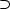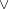#### 4.3 The program

##### 4.3.1 Operator definitions - syntax
 :- op(1100, xfy, implies).    :- op(1000, xfy, or).    :- op(900, xfy, and).    :- op(800, fy, ~).

or, and and implies are defined as infix operators.   is defined as a prefix operator. Note that these definitions are unusual in that they must be preceded by the :- operator. Omit this and you will get an error message to the effect that you are trying to redefine a system predicate. Defining a new operator consists of satisfying a goal which as a side-effect assigns the relevant precedence and associativity value to the operator.

##### 4.3.2 Operator definitions - semantics

or is defined as Prolog disjunction:

 or(X, Y):- X;Y.

and is defined as Prolog conjunction:

 and(X, Y) :- X, Y.

is defined as Prolog negation as failure:

 ~X :- \+ X.

The definition of implies is a little more cunning. The following is a standard tautology of propositional logic:

pq¬pq

as can be seen from the following truth table:

 (pq)((¬ p)q) t t t t f t t t t f f t f t f f f t t t t f t t f t t t t f t f

We can use this equivalence to define implies like this:

 implies(X, Y):- \+ X ; Y.

##### 4.3.3 The database

Declare p and q to be true.

 p.  q.

We assume that everything other than p and q is false, but we need to tell Prolog that we haven’t simply forgotten some of our definitions

 :- prolog_flag(unknown, _, fail).

This allows Prolog to simply fail if given an unknown predicate.

##### 4.3.4 The front end

The top-level predicate play/0 writes a prompt and accepts input with the built-in read/1 predicate, then evaluates the input.

evaluate/1 has three cases:

1. If X has the value ’stop’, then write ’goodbye’ and terminate.
 evaluate(X):-     X = stop,     write(goodbye), nl.

2. Call X and if it succeeds, write ’true’ and continue, via another call to play/0.
 evaluate(X):-     X,     write(true), nl,     play.

3. Otherwise, write ’false’ and continue.
 evaluate(_):-     write(false), nl,     play.

Finally, we insert a directive at the end of the file which Prolog will attempt to evaluate as soon as it consults it:

 :-play.

##### 4.3.5 Complete program listing
 /* file logic.pl */    /* A simple program to demonstrate the use of operators in Prolog.  Logical connectives and, or, if and ~ (negation) are defined as  operators and given the same semantics as their Prolog counterparts.  A small procedure play/0 is provided to allow the answers 'true' and  'false' to be supplied.  To run the program, type 'play.'  and then  input a propositional calculus expression, followed by a full stop and  carriage return.  e.g.     ?- play.  > |: p and q.  true  > p and r.  false  > r and r.  false  > q and r.  false  > q or r.  true  > p implies q.  true  > p implies q and r.  false  > p implies q or r.  true  > stop.  Goodbye    Type 'stop.' to finish. */    % Operator definitions    :- op(1000, xfy, or).    :- op(900, xfy, and).    :- op(800, fy, ~).    :- op(1100, xfx, implies).    % Allow Prolog to simply fail if given an unknown  % predicate    :- prolog_flag(unknown, _, fail).    % Definitions of connectives  ~X :- \+ X.  and(X, Y):-  X , Y.  or(X, Y):-   X ; Y.    % This one is cunning, 'X implies Y' is defined with  % the semantics of  (~X) or Y -- which has the same  % truth conditions.    implies(X, Y):- \+ X ; Y.    % p and q are true.  p.  q.    % Top level. Write a prompt and accept input. Then  % evaluate input.  play:-     write('> '),     read(X),     evaluate(X).    % If X is 'stop', then output 'goodbye' and terminate.  evaluate(X):-     X = stop,     write(goodbye), nl.    % If X succeeds, write 'true' and continue.  evaluate(X):-     X,     write(true), nl,     play.    % Otherwise, output 'false' and continue.  evaluate(_):-     write(false), nl,     play.    :-play.